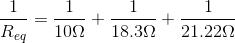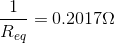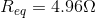High School Physics : Electricity and Magnetism

Example Questions

Example Question #1 : Understanding Voltage In A Circuit

A closed electric circuit is set up so that there is a current of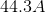and a resistance of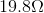. What is the voltage in the circuit?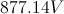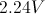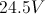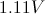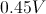Explanation:

For this problem, use Ohm's law: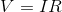.

We are given the current and the resistance. Using these terms, we can solve for the voltage.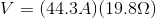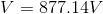Example Question #1 : Resistors

An electric circuit is set up in series with five resistors. If the resistors remain the same, but the circuit is now set up with the resistors in parallel, how would this affect the total resistance?

Resistance would increase substantially

Resistance would remain unchanged

Resistance would decrease substantially

We need to know the numerical values to solve

Resistance would decrease substantially

Explanation:

Think of resistors as doors, preventing the flow of people (electrons). Imagine the following scenarios: a large group of people are in a room and all try to leave at once. If the five resistors are in series, that's like having all of these people trying to go through all five doors before they can leave. In a circuit, all the electrons in the current mast pass through every resistor.

If the resistors are in parallel, it's like having five separate doors from the room. All of a sudden, the group can leave MUCH faster, encountering less resistance to their flow out of the room. The path of the electrons can split, allowing each particle to pass through only one resistor.

From a formula perspective, the resistors in series are simply summed together to find the equivalent resistance.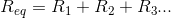In parallel, however, the reciprocals are summed to find the reciprocal equivalent resistance.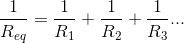Adding whole numbers will always give you a much greater result than adding fractions. For the exact same set of resistors, arrangement in series will have a greater total resistance than arrangement in parallel.

Example Question #31 : Electricity And Magnetism

When current in a circuit crosses a resistor, energy is lost. What form does this lost energy most commonly take?

The energy is converted into heat

The energy is converted into sound

The energy is converted into motion

The energy is converted into light

The energy is not converted; it simply disappears

The energy is converted into heat

Explanation:

In basic resistors, energy lost due to resistance is converted into heat. In some cases, other conversions also take place (such as generation of light in a lightbulb), but heat is still dissipated along with any alternative conversations. Lightbulbs, batteries, and other types of resistors will become hot as current passes through them.

Example Question #1 : Resistors

You have a 1m long, 0.5cm diameter copper wire that has an electric current running through it. Which of the following would decrease the wire's overall resistivity?

Decreasing the length of the wire

Increasing the length of the wire

Decreasing the diameter of the wire

None of these

Increasing the diameter of the wire

None of these

Explanation:

The formula for resistance is: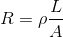Above,is length,is the cross-sectional area of the wire, and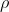is the resistivity of the material, and is a property of that material. The resistivity is constant for a given material, and thus cannot be changed by altering the dimensions of the wire.

Example Question #1 : Calculating Total Resistance

Ten resistors, each with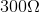resistance, are set up in series. What is their equivalent resistance?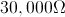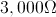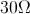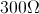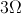Explanation:

For resistors aligned in series, the equivalent resistance is the sum of the individual resistances.Since all the resistors in this problem are equal, we can simplify with multiplication.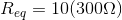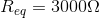Example Question #1 : Calculating Total Resistance

Ten resistors, each withresistance, are set up in parallel. What is the equivalent resistance?Explanation:

For resistors in parallel, the equivalent resistance can be found by summing the reciprocals of the individual resistances, then taking the reciprocal of the resultant sum.Since all the resistors in this problem are equal, we can simplify by multiplying.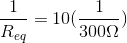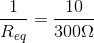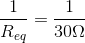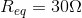Example Question #1 : Calculating Total Resistance

Twelve resistors of equal resistance are set up in series. If the total resistance is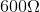, what is the resistance of each resistor?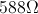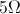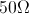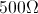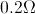Explanation:

The total resistance of resistors in series is the sum of the individual resistances.In this case, we know the total resistance and the number of resistors, and we are told that all resistors are of equal strength. That means we can simplify this problem using multiplication.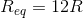We can use the total resistance to solve for the individual value.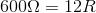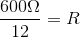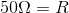Example Question #4 : Calculating Total Resistance

Three resistors are in a series. They have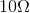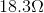, and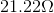of resistance respectively. What is the total resistance?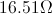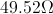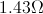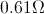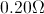Explanation:

When working in a series, the total resistance is the sum of the individual resistances.Use the given values for each individual resistor to solve for the total resistance.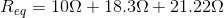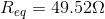Example Question #5 : Calculating Total Resistance

Six resistors are arranged in a series. Their resistances are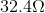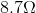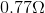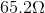, and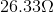. What is their total resistance?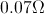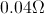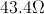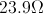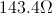Explanation:

When working in a series, the total resistance is the sum of the individual resistances.Use the given values for each individual resistor to solve for the total resistance.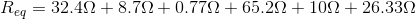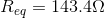Example Question #1 : Calculating Total Resistance

Three resistors are in a parallel circuit. They have resistances of, and, respectively. What is their total resistance?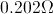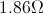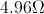Explanation:

The formula for resistors in parallel is:Using the given individual resistances, we can find the total resistance of the circuit.